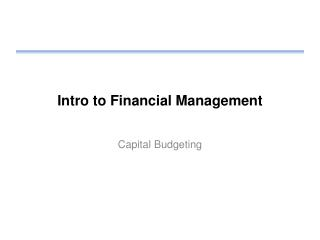DownloadDownload PresentationIntro to Financial Management

# Intro to Financial Management

Download Presentation## Intro to Financial Management

- - - - - - - - - - - - - - - - - - - - - - - - - - - E N D - - - - - - - - - - - - - - - - - - - - - - - - - - -
##### Presentation Transcript

1. Intro to Financial Management Capital Budgeting

2. Review • Homework • Cost of bonds • Use net proceeds • Use after-tax cost • Cost of common stock • Use net proceeds • Cost of retained earnings • Use CAPM

3. Capital Budgeting Should a project be accepted or rejected? How should a firm decide which projects to undertake?

4. Payback Period • Number of years to recover initial cash outlay • Example year1year2year3year4year5 Outlay -\$10k CashFlow \$2k \$4k \$3k \$3k \$9k • Payback is 3.33 years • Firm may set criteria, such as 3 year payback period • In this case, would reject the project

5. Payback Period Positives • Deals with cash flows, not profits • Easy to calculate Negatives • Requires good forecasts • Ignores time value of money • Completely ignores money after payback period threshold • Payback period may be arbitrary

6. Discounted Payback • Instead of using simple cash flows – use discounted cash • Then calculate the number of years to payback • Payback period requirement is still arbitrary

7. Net Present Value MethodNPV • NPV – the net of all future discounted cash flows • Includes entire life of project • Later years will be discounted greater • Remember to discount using cost of capital (hurdle rate), k • Can deal with outlays in future years NPV = PV(benefits) – PV(costs) • NPV shows today’s value of a project • Decision criteria is simple • If NPV > 0, accept the proposal

8. Profitability Index Method(Benefit – Cost Ratio) • PI = PV(future cash) / initial outlay • Decision criteria is simple • If PI > 1, accept the proposal • Similar to NPV • But only uses initial outlay • In basic form does not accommodate future outlays unless factored into future cash flows

9. Internal Rate of Return (IRR) • Instead of using k for the discount factor, compute the IRR on the calculator • 2nd CLR WORK <clears old data> • CF -5000 Enter <the initial outlay> • ↓ 2000 Enter <first cash flow> • ↓ 2 Enter <# periods for prior cash flow> • ↓ 3000 Enter <next cash flow> • ↓ 1 Enter <# periods for prior cash flow> • IRR CPT <Note: press CPT after pressing IRR> 17.50% IRR • Decision criteria • Accept of IRR > hurdle rate (cost of capital)

10. NPV – IRR Relationship • The higher the discount rate, the lower the NPV • IRR is discount rate where NPV = 0 • Can have situation with multiple solutions to IRR • Get when future flows may be negative • IRR is then meaningless • Use NPV

11. Modified IRR (MIRR) • Calculate the PV of all cash outflows • Use k for discount rate • Calculate the FV of all cash inflows • Use k for discount rate • Result called terminal value, TV • Calculate the MIRR Find the discount rate that equates 1 and 2 PV(outflows) = TV(inflows) / (1 + MIRR)n Calculate on calculator using CPT I/Y • Accept if MIRR > hurdle rate

12. MIRR Example • See earlier IRR example • Assume cost of capital = 8% • Cash flows look like: -5000 2000 2000 3000 • Compute PV of outflows • Is just -5000 • Compute FV of inflows • 2000 for 2 years = 2,332.80 • 2000 for 1 year = 2,160 • 3000 in last year • Total of 7,492.80 • Now compute IRR PV = -5000, FV = 7,492.80, N = 3, CPT I/Y = MIRR = 14.43

13. Capital Rationing • Have limits on capital available for investing • Can not accept all projects • Rank by NPV and accept those with the most value to the business • Maximizes shareholder wealth Practical concern • May want to consider discounted payback method as a way to evaluate risk • Two project may both have NPV > 0 • Longer payback means more risk in something going wrong